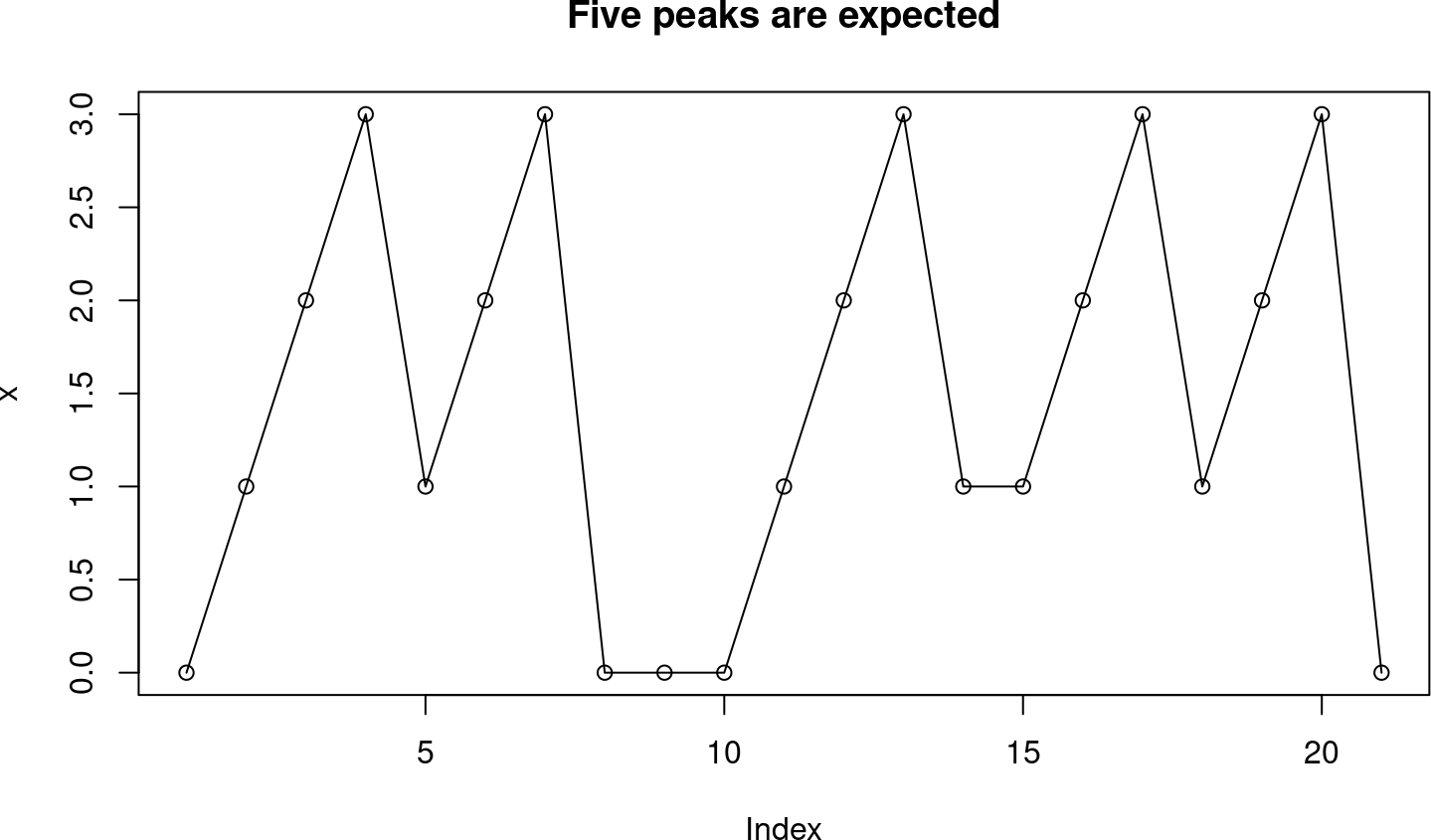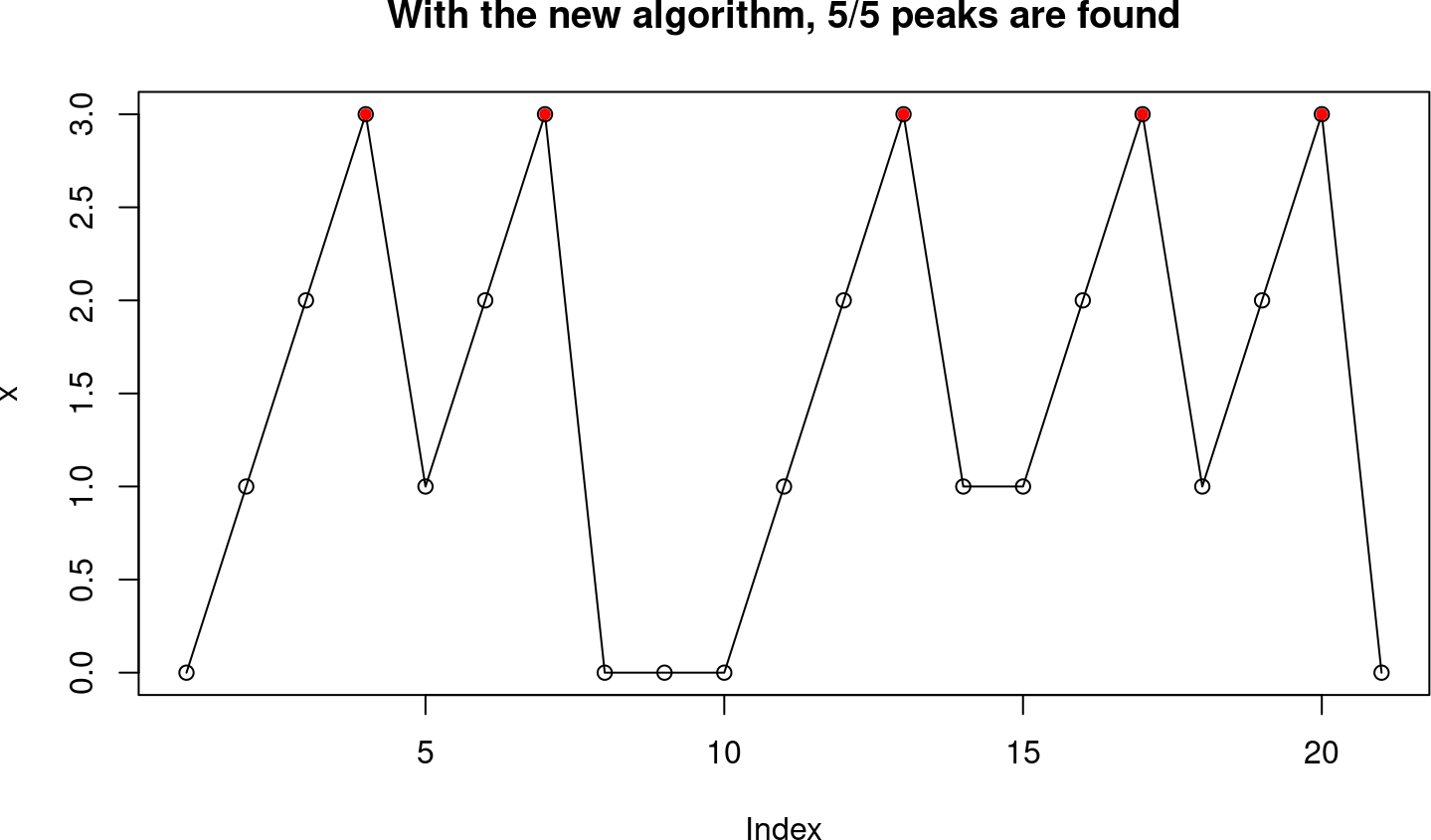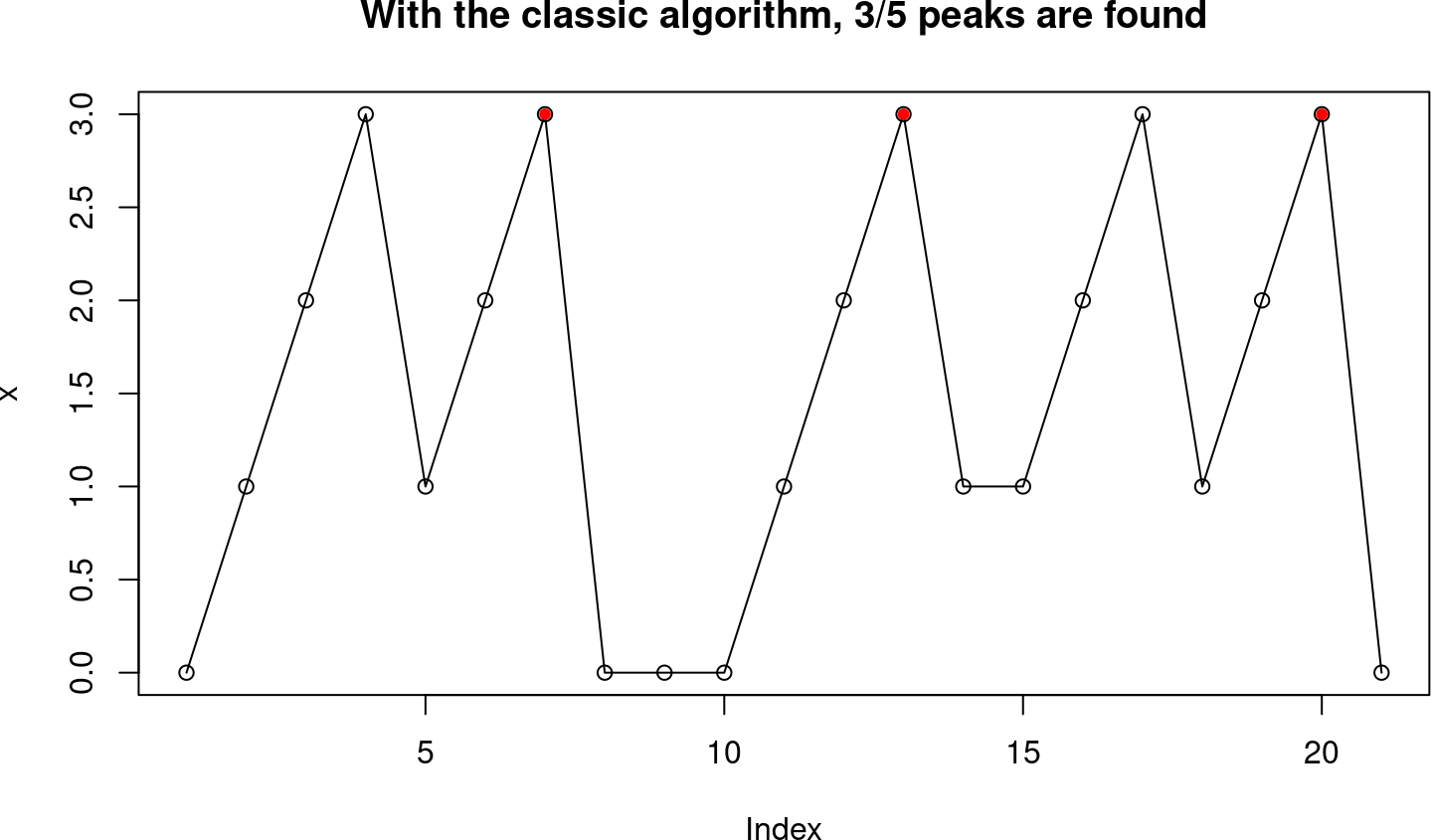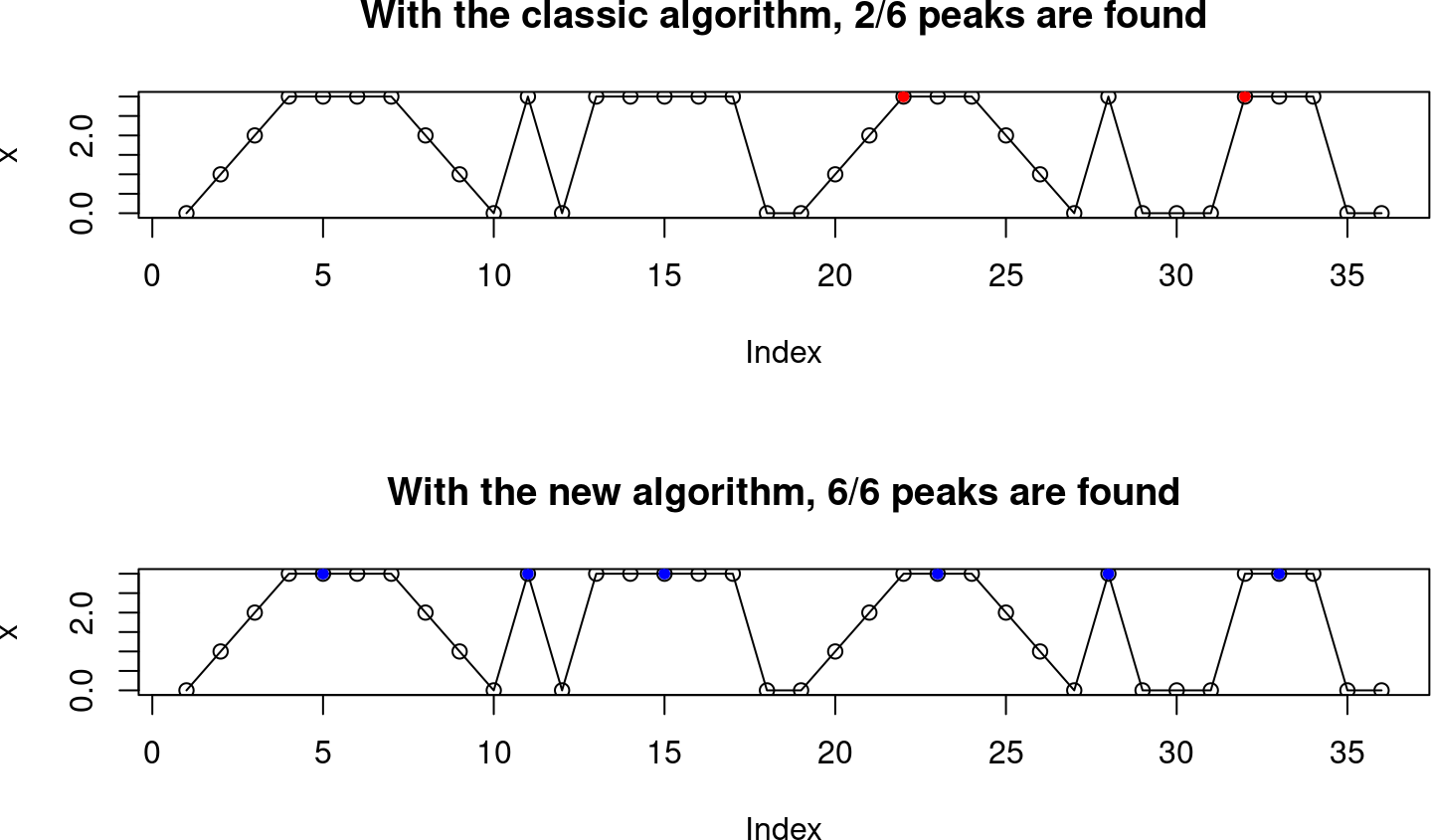# 1 Introduction

As described in the introductory vignette, one of the steps of the peak detection process in the MassSpecWavelet package relies on the detection of local maxima on the wavelet coefficients.

On some benchmarks (not shown), this local maxima detection step accounted for the largest computational cost of the MassSpecWavelet peak detection algorithm.

MassSpecWavelet version 1.63.3 provides three algorithms for detecting local maxima, named “classic”, “faster” and “new”.

• “classic” is the algorithm found in previous versions. As a reference implementation.
• “faster” is a new and faster implementation of the “classic” algorithm. It gives identical results to “classic” in less time. It’s the default algorithm.
• “new” is a new algorithm for local maxima detection, that has different behaviour and therefore gives different results. It is experimental and not used by default.

You can switch between the algorithms by setting the `"MassSpecWavelet.localMaximum.algorithm"` option as follows:

``options("MassSpecWavelet.localMaximum.algorithm" = "classic")``

In a future version, we may remove “classic”, replacing it with “faster” since they both use the exact same criteria. The algorithm option is experimental, and in future versions we may change the API or the algorithm names. While the MassSpecWavelet package has a very stable API, this stability does not apply to this option. Feedback is very much welcome on https://github.com/zeehio/MassSpecWavelet/issues/.

The `"new"` algorithm addresses some issues found in the “classic” algorithm, as well as in its “faster” implementation. In this vignette:

• We show the issues of the classic `localMaximum()` detection algorithm
• We describe the new algorithm
• We compare the required CPU time of the three implementations

We are still exploring the impact of this new `localMaxima()` algorithm on the peak detection pipeline provided by MassSpecWavelet. For now, the peak detection pipeline uses the `"faster"` algorithm.

If you want to try using the `"new"` local maxima algorithm within the peak detection pipeline, you can use `options("MassSpecWavelet.localMaximum.algorithm" = "new")`. Consider that experimental though.

``library(MassSpecWavelet)``

# 2 Problems with the classic algorithm

The `"classic"` `localMaximum` algorithm implements the local maxima detection using a two partially overlapping non-sliding windows: One that starts at the beginning of the signal, and another one starting at half the window size.

The documentation reflected this behavior in the Details section:

Instead of find the local maximum by a slide window, which slide all possible positions, we find local maximum by transform the vector as matrix, then get the the maximum of each column. This operation is performed twice with vecctor shifted half of the winSize. The main purpose of this is to increase the efficiency of the algorithm.

While it’s true that this makes the algorithm faster, it does so at the expense of missing some local maxima under some conditions. See for instance the following artificial signal:

``````onetwothree <- c(1,2,3)
x <- c(0, onetwothree, onetwothree, 0, 0, 0, onetwothree, 1, onetwothree, onetwothree, 0)
plot(x, type = "o", main = "Five peaks are expected")``````If we use a sliding window of four points we can detect the five peaks (if you actually look at each maximum you will be able to draw some window that contains the maximum and it has at least four points inside). So when we use the new algorithm:

``````options("MassSpecWavelet.localMaximum.algorithm" = "new")
local_max <- which(localMaximum(x, winSize = 4) > 0)
plot(x, type = "o", main = "With the new algorithm, 5/5 peaks are found")
points(local_max, x[local_max], col = "red", pch = 20)``````However with the classic algorithm, there are two peaks we are missing:

``````options("MassSpecWavelet.localMaximum.algorithm" = "classic")
local_max <- which(localMaximum(x, winSize = 4) > 0)
plot(x, type = "o", main = "With the classic algorithm, 3/5 peaks are found")
points(local_max, x[local_max], col = "red", pch = 20)``````While this is less likely to happen with longer window sizes, and the default window size in the peak detection is `2*scale+1`, this efficiency shortcut may have some actual impact on the peak detection of MassSpecWavelet.

I haven’t measured how often this happens in real mass spectrometry samples. Feel free to compare the results and get back to me, I guess the impact will be rather small, otherwise this issue would have been noticed earlier.

Benchmarking the performance of the MassSpecWavelet peak detection algorithm, this local maximum detection was also the most computationally intensive part in my tests, so finding a more efficient (and more correct) algorithm seemed a worth pursuing goal to improve the package.

# 3 The new local maximum algorithm

We want an algorithm to detect local maximum using a sliding window. Since we are putting effort in correctness, we want to define it properly.

A point in the signal will be reported as a local maximum if one of these conditions are met:

• We can draw some window of size `winSize` that (a) contains our point (b) our point is not in the border of the window and (c) is the largest value of the window.

• If a point is part of a plateau of constant values, the center of the plateau will be considered as the maximum. If the plateau has an even size, it will be the first of the two points that could be the center of the plateau.

To give an example of these plateaus (very unlikely to happen with floating point values) see this synthetic example and how the two algorithms behave:

``````x <- c(0, 1, 2, 3, 3, 3, 3, 2, 1, 0, 3, 0, 3, 3, 3, 3, 3, 0)
x <- c(x, 0, 1, 2, 3, 3, 3, 2, 1, 0, 3, 0, 0, 0, 3, 3, 3, 0, 0)
options("MassSpecWavelet.localMaximum.algorithm" = "classic")
local_max_classic <- which(localMaximum(x, winSize = 5) > 0)
options("MassSpecWavelet.localMaximum.algorithm" = "new")
local_max_new <- which(localMaximum(x, winSize = 5) > 0)
par(mfrow = c(2, 1))
plot(x, type = "o", main = "With the classic algorithm, 2/6 peaks are found")
points(local_max_classic, x[local_max_classic], col = "red", pch = 20)
plot(x, type = "o", main = "With the new algorithm, 6/6 peaks are found")
points(local_max_new, x[local_max_new], col = "blue", pch = 20)``````While these plateaus are corner cases in real scenarios very unlikely to happen, it is worth having a well-defined and well-behaved implementation.

# 4 Computational cost

Figure 1 shows the computational time to find the local maxima using the `"new"` algorithm and the `"classic"` algorithm (in both the `"classic"` and the `"faster"` implementations).

``````# Run this interactively
set.seed(5413L)
winSizes <- c(5, 31, 301)
xlengths <- c(20, 200, 2000, 20000, 200000)
out <- vector("list", length(winSizes) * length(xlengths))
i <- 0L
for (winSize in winSizes) {
for (xlength in xlengths) {
i <- i + 1L
x <- round(10*runif(xlength), 1)*10
bm1 <- as.data.frame(
bench::mark(
classic = {
options(MassSpecWavelet.localMaximum.algorithm = "classic")
localMaximum(x, winSize = winSize)

},
faster = {
options(MassSpecWavelet.localMaximum.algorithm = "faster")
localMaximum(x, winSize = winSize)

},
check = TRUE,
filter_gc = FALSE,
time_unit = "ms"
)
)
bm2 <- as.data.frame(
bench::mark(
new = {
options(MassSpecWavelet.localMaximum.algorithm = "new")
localMaximum(x, winSize = winSize)

},
check = FALSE,
filter_gc = FALSE,
time_unit = "ms"
)
)
out[[i]] <- data.frame(
algorithm = c(as.character(bm1\$expression), as.character(bm2\$expression)),
min_cpu_time_ms = c(as.numeric(bm1\$min), as.numeric(bm2\$min)),
xlength = xlength,
winSize = winSize
)
}
}
out2 <- do.call(rbind, out)
plot(
x = out2\$xlength,
y = out2\$min_cpu_time_ms,
col = ifelse(out2\$algorithm == "new", "red", ifelse(out2\$algorithm == "faster", "darkgreen", "blue")),
pch = ifelse(out2\$winSize == 5, 1, ifelse(out2\$winSize == 31, 2, 3)),
log = "xy",
xlab = "Signal length",
ylab = "Min CPU time (ms)"
)
legend(
"topleft",
legend = c("New algorithm", "Classic algorithm", "Faster algorithm",
"winSize = 5", "winSize = 31", "winSize = 301"),
lty = c(1,1,1, 0, 0, 0),
pch = c(NA, NA, NA,1, 2, 3),
col = c("red", "blue", "darkgreen", "black", "black", "black")
)``````